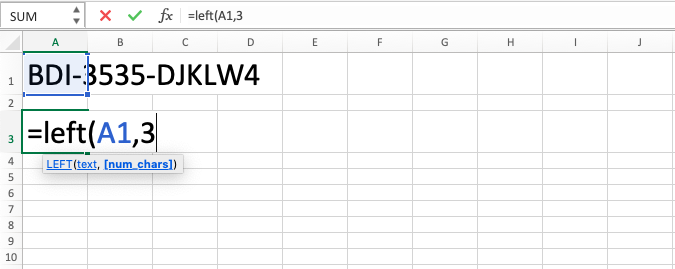LEFT Function in Excel - Compute Expert

# LEFT Function in Excel

In this tutorial, you will understand how to use LEFT formula in excel. LEFT function in excel is useful to separate characters from data or content of a cell in the spreadsheet software.

## Why We Need to Learn About LEFT Formula in Excel?

In the data owned and saved in spreadsheet tool, sometimes we need to take some characters from the data with the starting position in the front. It is so that we can use these in our data analysis.

For example, if we need just the date data from a group of date, month, and year that we have in a column on this spreadsheet software. This kind of thing might be needed to analyze product sales trend in a month as a basis to take important decision for marketing communication schedule from the company marketing division. To get that for the company needs, we may want to do it manually to take the data from that column. But, if the data is a lot or dynamically changed, we will spend a lot of time if there is no automatic way to do it.

For solving that problem, we can use LEFT function in excel to take characters from the front on the data that we have in spreadsheet software. LEFT formula in excel can help us separate characters according to our needs by specifying the data or cell where the characters will be taken and also how many characters that we want to take from the front of the data.

## What is LEFT Function in Excel?

LEFT function in excel is a formula that can be used to take characters according to our needs from the front starting position of a data/cell content. This LEFT formula in excel is different from other characters separating formulas in this spreadsheet software such as MID and RIGHT in the starting position determination to take the characters.

A little bit of explanation on the inputs in this formula is as follows:

=LEFT(text, num_chars)

Note:
• text = the data where some of the characters will be taken from
• num_chars = the amount of characters that you want to take from the most left position of the data

## How to Use LEFT Formula in Excel?

The next part will explain how to use LEFT function in excel step by step. If you have known how to use RIGHT formula before, then the writing of this LEFT formula in excel is pretty similar to that.

## Using LEFT Function in Excel

1. Type equal sign ( = ) in the cell where you want to put the result in2. Type LEFT (can be with large and small letters) and open bracket sign after =3. Type data or cell coordinate where the data which you want to process with this formula is after open bracket sign followed by a comma sign ( , )4. Type the number to represent how many characters will you take from the most left/cell coordinate where the number is after comma sign5. Type close bracket sign6. Press Enter
7. The process is done!## Exercise

After you learn how to use LEFT formula in excel, you can practice your understanding through the exercise below!

### Questions

1. Take the date part from the question in column B!
2. Take the first name from the whole name of the person in column C!
3. Take all the numbers before going to the decimal point in column D!

There are other characters’ separation formulas in this spreadsheet software such as MID and RIGHT. The difference with the formula explained here, as being told previously, is in the starting position determination for the character separation from your data/cell content. If you want, you can learn them also from other parts of this Compute Expert blog!

Get updated excel info from Compute Expert by registering your email. It's free!